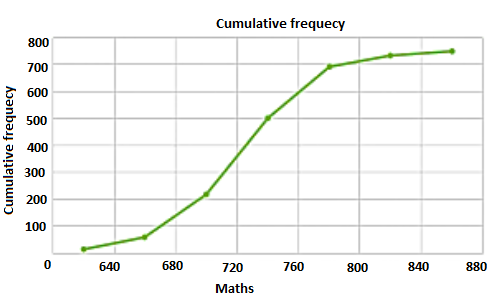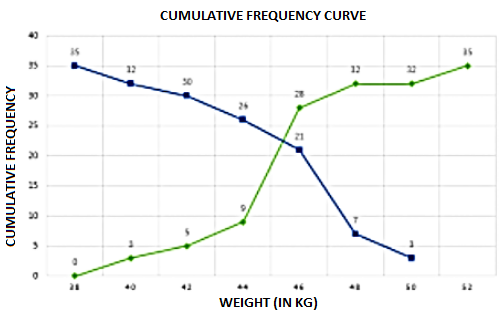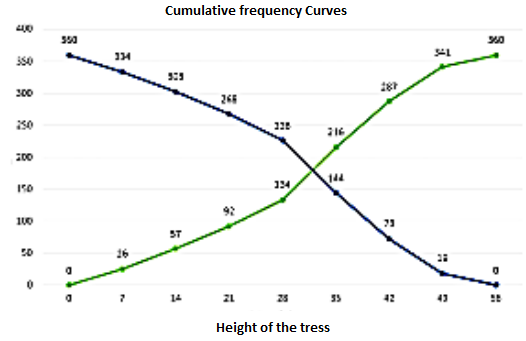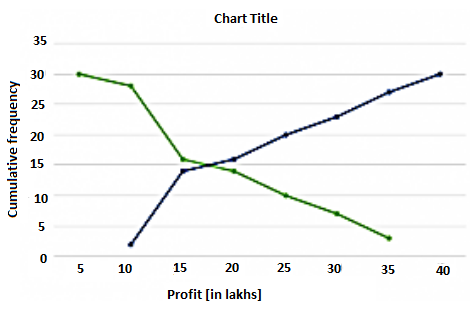×#### Thank you for registering.

One of our academic counsellors will contact you within 1 working day.

Click to Chat

1800-1023-196

+91-120-4616500

CART 0

• 0

MY CART (5)

Use Coupon: CART20 and get 20% off on all online Study Material

ITEM
DETAILS
MRP
DISCOUNT
FINAL PRICE
Total Price: Rs.

There are no items in this cart.
Continue Shopping• Complete JEE Main/Advanced Course and Test Series
• OFFERED PRICE: Rs. 15,900
• View Details

```Chapter 7: Statistics Exercise – 7.6

Question: 1

Draw an Ogive by less than the method for the following data:

No. of rooms
No. of houses

1
4

2
9

3
22

4
28

5
24

6
12

7
8

8
6

9
5

10
2

Solution:

No. of rooms
No. of houses
Cumulative Frequency

Less than or equal to 1
4
4

Less than or equal to 2
9
13

Less than or equal to 3
22
35

Less than or equal to 4
28
63

Less than or equal to 5
24
87

Less than or equal to 6
12
97

Less than or equal to 7
8
107

Less than or equal to 8
6
113

Less than or equal to 9
5
119

Less than or equal to 10
2
120

We need to plot the points (1, 4), (2, 3), (3, 35), (4, 63), (5, 87), (6, 99), (7, 107), (8, 113), (9, 118), (10, 120), by taking upper class limit over the x-axis and cumulative frequency over the y-axis.Question: 2

The marks scored by 750 students in an examination are given in the form of a frequency distribution table:

Marks
No. of Students

600 – 640
16

640 – 680
45

680 – 720
156

720 – 760
284

760 – 800
172

800 – 840
59

840 – 880
18

Solution:

Marks
No. of Students
Marks Less than
Cumulative Frequency

600 – 640
16
640
16

640 – 680
45
680
61

680 – 720
156
720
217

720 – 760
284
760
501

760 – 800
172
800
693

800 – 840
59
840
732

840 – 880
18
880
750

Plot the points (640, 16), (680, 61), (720, 217), (760, 501), (800, 673), (840, 732), (880, 750) by taking upper class limit over the x-axis and cumulative frequency over the y-axis.Question: 3

Draw an Ogive to represent the following frequency distribution:

Class-interval
0 – 4
5 – 9
10 – 14
15 – 19
20 – 24

No. of students
2
6
10
5
3

Solution:

The given frequency distribution is not continuous, so we will first make it continuous and then prepare the cumulative frequency:

Class-interval
No. of Students
Less than
Cumulative frequency

0.5 – 4.5
2
4.5
2

4.5 – 9.5
6
9.5
8

9.5 – 14.5
10
14.5
18

14.5 – 19.5
5
19.5
23

19.5 – 24.5
3
24.5
26

Plot the points (4.5, 2), (9.5, 8), (14.5, 18), (19.5, 23), (24.5,26) by taking the upper class limit over the x-axis and cumulative frequency over the y-axis.Question: 4

The monthly profits (in Rs) of 100 shops are distributed as follows:

Profit per shop
No of shops:

0 – 50
12

50 – 100
18

100 – 150
27

150 – 200
20

200 – 250
17

250 – 300
6

Draw the frequency polygon for it

Solution:

We have

Profit per shop
Mid-value
No of shops:

Less than 0
0
0

Less than 0 – 60
25
12

Less than 60 – 120
75
18

Less than 120 – 180
125
27

Less than 180 – 240
175
20

Less than 240 – 300
225
17

Less than 300 – 360
275
6

Above 360
300
0Question: 5

The following distribution gives the daily income of 50 workers of a factory:

Daily income (in Rs):
No of workers:

100 – 120
12

120 – 140
14

140 – 160
8

160 – 180
6

180 –  200
10

Convert the above distribution to a 'less than' type cumulative frequency distribution and draw its ogive.

Solution:

We first prepare the cumulative frequency table by less than method as given below:

Daily income
Cumulative frequency

<120
12

<140
26

<160
34

<180
40

<200
50

Now we mark on x-axis upper class limit, y-axis cumulative frequencies. Thus we plot the point (120, 12), (140, 26), (160, 34), (180, 40), (200, 50).Question: 6

The following table gives production yield per hectare of wheat of 100 farms of a village:

Production yield:
No of farms:

50 – 55
2

55 – 60
8

60 – 65
12

65 – 70
24

70 – 75
38

75 – 80
16

Draw 'less than' ogive and 'more than' ogive

Solution:

Less than method: Cumulative frequency table by less than method

Production yield
Number of farms
Production yield more than
Cumulative frequency

50 – 55
2
55
2

55 – 60
8
60
10

60 – 65
12
65
22

65 – 70
24
70
46

70 – 80
38
75
84

75 – 80
16
80
100

Now we mark on x-axis upper class limit, y-axis cumulative frequencies. We plot the point (50,100), (55, 98), (60, 90), (65, 78), (70, 54), (75, 16)Question: 7

During the medical check-up of 35 students of a class, their weight recorded as follows:

Weight (in kg)
No of students

Less than 38
0

Less than 40
3

Less than 42
5

Less than 44
9

Less than 46
14

Less than 48
28

Less than 50
32

Less than 52
35

Draw a less than type ogive for the given data. Hence, obtain the median weight from the graph and verify the verify the result my using the formula.

Solution:

Less than method: It is given that On x-axis upper class limits. Y-axis cumulative frequency We plot the points (38, 0), (40, 3), (42, 5), (44, 9), (46, 4), (48, 28), (50, 32), (52, 35).

More than method: cumulative frequency

Weight
No. of students
Weight more than
Cumulative frequency

38 – 40
3
38
34

40 – 42
2
40
32

42 – 44
4
42
30

44 – 46
5
44
26

46 – 48
14
46
21

48 – 50
4
48
7

50 – 52
3
50
3

x – axis lower class limits on y-axis cf We plot the points (38, 35), (40, 32), (42, 30), (44, 26), (46, 26), (48, 7), (50,3).Question: 8

The following table shows the height of trees:

Height
No. of trees

Less than  7
26

Less than  14
27

Less than  21
92

Less than  28
134

Less than  35
216

Less than  42
287

Less than  49
341

Less than  56
360

Draw 'less than' ogive and 'more than' ogive

Solution:

By less than method

Height
No. of trees

Less than  7
26

Less than  14
27

Less than  21
92

Less than  28
134

Less than  35
216

Less than  42
287

Less than  49
341

Less than  56
360

Plot the points (7, 26), (14, 57), (21, 92), (28, 134), (35, 216), (42, 287), (49, 341), (56, 360) by taking upper class limit over the x-axis and cumulative frequency over the y-axis.

By more than method:

Height
No. of trees
Height more than
C.F.

0 – 7
26
0
360

7 – 14
27
5
334

14 – 21
92
10
303

21 – 28
134
15
268

28 – 35
216
20
226

35 – 42
287
25
144

42 – 49
341
30
73

49 – 56
360
35
19Question: 9

The annual profits earned by 30 shops of a shopping complex in a locality give rise to the following distribution:

Profit (In lakhs In Rs)
Number of shops (frequency)

More than or equal to 5
30

More than or equal to 10
28

More than or equal to 15
16

More than or equal to 20
14

More than or equal to 25
10

More than or equal to 30
7

More than or equal to 35
3

Draw both ogive for the above data and hence obtain the median.

Solution:

More than method:

Profit (In lakhs In Rs)
Number of shops (frequency)

More than or equal to 5
30

More than or equal to 10
28

More than or equal to 15
16

More than or equal to 20
14

More than or equal to 25
10

More than or equal to 30
7

More than or equal to 35
3

Now, we mark on x-axis lower class limits, y-axis cumulative frequency. Thus, we plot the points (5, 30), (10, 28), (15, 16), (20, 14), (25,10), (30, 7) and (35, 3)

Less than method:

Profit in lakhs
No of shops
Profits less than
C.F

0 – 10
2
10
2

10 – 15
12
15
14

15 – 20
2
20
16

20 – 25
4
25
20

25 – 30
3
30
23

30 – 35
4
35
27

35 – 40
3
40
30

Now we mark the upper class limits along x-axis and cumulative frequency along y-axis. Thus we plot the points (10, 2), (15,14), (20,16), (25, 20), (30, 23), (35, 27), (40, 30).

We find that the two types of curves intersect of P from point L it is drawn on x-axis The value of a profit corresponding to M is 17.5. Hence median is 17.5 lakh```### Course Features

• 728 Video Lectures
• Revision Notes
• Previous Year Papers
• Mind Map
• Study Planner
• NCERT Solutions
• Discussion Forum
• Test paper with Video Solution